# NCERT Solutions class-11 Chemistry States of Matter## myCBSEguide App

Complete Guide for CBSE Students

NCERT Solutions, NCERT Exemplars, Revison Notes, Free Videos, CBSE Papers, MCQ Tests & more.

States of matter
1. What will be the minimum pressure required to compress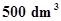of air at 1 bar to 200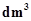at 30°C?

2. A vessel of 120 mL capacity contains a certain amount of gas at 35 °C and 1.2 bar pressure. The gas is transferred to another vessel of volume 180 mL at 35 °C. What would be its pressure?

3. Using the equation of state pV = nRT; show that at a given temperature density of a gas is proportional to gas pressure p.

4. At 0°C, the density of a certain oxide of a gas at 2 bar is same as that of dinitrogen at 5 bar. What is the molecular mass of the oxide?

5. Pressure of 1 g of an ideal gas A at 27 °C is found to be 2 bar. When 2 g of another ideal gas B is introduced in the same flask at same temperature the pressure becomes 3 bar. Find a relationship between their molecular masses.

6. The drain cleaner, Drainex contains small bits of aluminum which react with caustic soda to produce dihydrogen. What volume of dihydrogen at 20 °C and one bar will be released when 0.15g of aluminum reacts?

7. What will be the pressure exerted by a mixture of 3.2 g of methane and 4.4 g of carbon dioxide contained in a 9flask at 27 °C ?

8. What will be the pressure of the gaseous mixture when 0.5 L of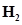at 0.8 bar and 2.0 L of dioxygen at 0.7 bar are introduced in a 1L vessel at 27°C?

9. Density of a gas is found to be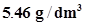at 27 °C at 2 bar pressure. What will be its density at STP?

10. 34.05 mL of phosphorus vapour weighs 0.0625 g at 546 °C and 0.1 bar pressure. What is the molar mass of phosphorus?

11. A student forgot to add the reaction mixture to the round bottomed flask at 27 °C but instead he/she placed the flask on the flame. After a lapse of time, he realized his mistake, and using a pyrometer he found the temperature of the flask was 477 °C. What fraction of air would have been expelled out?

12. Calculate the temperature of 4.0 mol of a gas occupying 5at 3.32 bar.

(R = 0.083 bar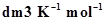).

13. Calculate the total number of electrons present in 1.4 g of dinitrogen gas.

14. How much time would it take to distribute one Avogadro number of wheat grains, if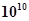grains are distributed each second?

15. Calculate the total pressure in a mixture of 8 g of dioxygen and 4 g of dihydrogen confined in a vessel of 1at 27°C. R = 0.083 bar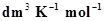.

Ans. Given,

16. Pay load is defined as the difference between the mass of displaced air and the mass of the balloon. Calculate the pay load when a balloon of radius 10 m, mass 100 kg is filled with helium at 1.66 bar at 27°C. (Density of air = 1.2 kg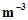and R = 0.083 bar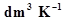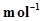).

17. Calculate the volume occupied by 8.8 g of CO2 at 31.1°C and 1 bar pressure.

R = 0.083 bar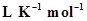.

18. 2.9 g of a gas at 95 °C occupied the same volume as 0.184 g of dihydrogen at 17 °C, at the same pressure. What is the molar mass of the gas?

19. A mixture of dihydrogen and dioxygen at one bar pressure contains 20% by weight of dihydrogen. Calculate the partial pressure of dihydrogen.
20. What would be the SI unit for the quantity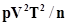?
21. In terms of Charles’ law explain why -273°C is the lowest possible temperature.
22. Critical temperature for carbon dioxide and methane are 31.1 °C and -81.9 °C respectively. Which of these has stronger intermolecular forces and why?
23. Explain the physical significance of Van der Waals parameters.## Test Generator

Create Papers with your Name & Logo